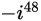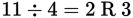# SAT Math Multiple Choice Question 945: Answer and Explanation

### Test Information

Question: 945

13. What is the value of?

• A. -i
• B. i
• C. -1
• D. 1

Strategic Advice: Powers of i cycle in the pattern i, -1, -i, 1. Memorizing this pattern may save you valuable time on Test Day. To determine the value of a high power of i, divide the exponent by 4 (the number of terms in the cycle). The remainder tells you which term in the pattern is the answer. For example, i11 = i3 because.